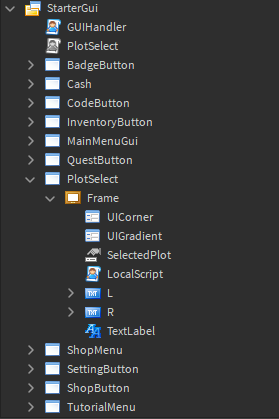# Cannot Enable a script in PlayerGui

1. What do you want to achieve?
Fix the script and let it work.

2. What is the issue?
Disabled is not a valid member of ScreenGui “Players.superender11111.PlayerGui.PlotSelect”
cannot enable a localscript in PlayerGui
my script:

``````task.wait(10)

script.Parent.Visible = true

game.Players.LocalPlayer.PlayerGui.PlotSelect.Disabled = false
game.StarterGui.PlotSelect.Disabled = false
end
``````

my starterGui:the PlotSelect script:

``````local TweenService = game:GetService("TweenService")

local Player = game.Players.LocalPlayer
local Camera = game.Workspace.Camera

local PlotSelect = script.Parent:WaitForChild("PlotSelect")

local Frame = PlotSelect:WaitForChild("Frame")
local Left = Frame:WaitForChild("L")
local Right = Frame:WaitForChild("R")

local SelectedPlot = Frame:WaitForChild("SelectedPlot")

local Plots = game.Workspace.Simworld.plot

Camera.CameraType = Enum.CameraType.Scriptable

local function findUnoccupiedPlots()
local availablePlots = {}
for i, plot in pairs(Plots:GetChildren()) do
if plot.Occupant.Value == nil then
table.insert(availablePlots,plot)
end
end
return availablePlots

end

local TI = TweenInfo.new(
0.5,
Enum.EasingStyle.Quint,
Enum.EasingDirection.InOut,
0,
false,
0
)

local function camTween(plot)
local cf = CFrame.new(plot.Position+Vector3.new(0,120,0),plot.Position)
local tween = TweenService:Create(game.Workspace.Camera,TI,{CFrame = cf})
tween:Play()
end

local plotsTable = findUnoccupiedPlots()

local index = 1

SelectedPlot.Value = plotsTable
camTween(SelectedPlot.Value)

Right.MouseButton1Click:Connect(function()
if Plots:FindFirstChild("Plot" .. index-1) then
index -= 1
else

index = 6
end

SelectedPlot.Value = plotsTable[index]
camTween(plotsTable[index])
end)

Left.MouseButton1Click:Connect(function()
if Plots:FindFirstChild("Plot" .. index+1) then
index += 1
else
index = 1
end

SelectedPlot.Value = plotsTable[index]
camTween(plotsTable[index])
end)
``````
1. What solutions have you tried so far?
i tried to make disable=false in StarterGui but the PlotSelect script didnt run.
i am new to scripting so pls help.This is refering to the ScreenGui named “PlotSelect”. ScreenGuis only have an “Enabled” property

BUT if you’re trying to reference the localscript then it should be `game.Players.LocalPlayer.PlayerGui.PlotSelect.Frame.LocalScript`

LocalScripts have a “Disabled” property, so you can do `.Disabled` on this.

1 Like

thank you, you reminded me i have two children name PlotSelect.

1 Like